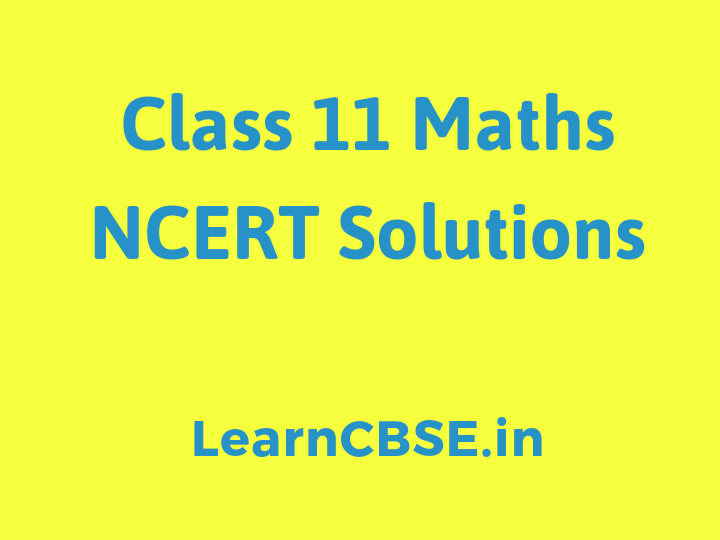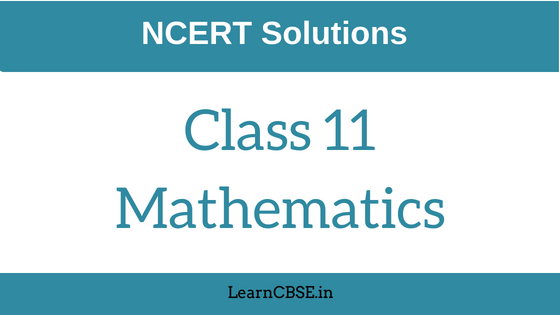NCERT Solutions for Class 11 Maths consist of solved answers for all the chapters, exercise-wise. This is a great material for students who are preparing for Class 11 exams. The solutions provided here are with respect to NCERT syllabus and curriculum. These materials are prepared by our expertise keeping on mind students understanding level.

## Class 11 Maths NCERT Solutions

NCERT solutions for 11th standard Maths subject covers the chapters which are present in the textbook, exercise-wise. The chapters covered here are Sets, Trigonometric functions, sequences and series, Three dimensional geometry, Relations and functions, statistics, binomial theorem, probability, etc. The solutions for these chapters are available in PDF format, which students can download easily and learn them as per their convenience.

NCERT textbooks are prescribed by CBSE board. So all the students who are studying under this board have the best solution materials here. Class 11 is also an important class for students, as the level of education increases here. In this standard, students have opted for Maths subject as their main subject. Students have to more focussed about their studies as this is the start of their future learning and career. Therefore, using these materials students can really score good marks in their final exams.

All the solutions for all the chapters for class 11 Maths subject have been arranged in a proper manner. Students can easily find answers for any question here which is mentioned in the book. These solutions are in accordance with 2019-2020 syllabus. So students need not worry about the given content for the solutions. They have well prepared and structured solutions available here, by us.### NCERT Class 11 Maths Solutions

Solving the questions present in the textbooks is not an easy task. It requires mathematical and logical skills to solve those problems with a lot of efforts. Class 11th Maths is a next level Maths, where students will learn a large variety of topics, which they are going to face in higher studies as well, such as in Class 12th.

As we all know, 12th is the most important class for all the students, as after this they move to their college level. But you can get admission in a good recognized institute or university, only when you have score the minimum qualifying marks. And it is only then, you will be able to get good jobs or get placed directly from campus recruitment, conducted by colleges. Therefore, it very necessary for you to build a strong base in class 11 maths subject and understand the concepts deeply.

To make it easier for them to learn, we are providing here solutions for all the students of 11th standard, such that, they can clarify their doubts for all types of questions. Below are the introductions for each chapter and links for all the exercises, read them thoroughly.

In this Chapter, we will study about this approach called axiomatic approach of probability. To understand this approach we must know about few basic terms viz. random experiment, sample space, events, etc.

We have tried to give the best solutions for all the chapters of NCERT textbooks Maths for class 11. If students finds here any difficulty, please drop a comment, we will try to resolve them as soon as possible.

### Which book is best for class 11 maths?

NCERT Class 11 Math Textbook is best and enough for CBSE Class 11 Exam preparation. RD Sharma class 11 Textbook is best reference book for Class 11 students.

### How many chapters are there in class 11 maths?

There are 16 chapters starting from Sets to Probability. LearnCBSE.in has included all the chapters Answers in this page.

### Is class 11 Maths tough?

No. If you have very good foundation in lower classes, Maths will be very easy. CBSE has designed curriculum based on NCERT guidelines. These NCERT Textbooks are very good in presenting concepts and application based on these concepts. LearnCBSE.in recommends you to study introduction part of each and every chapter get the firm grip on the concepts.